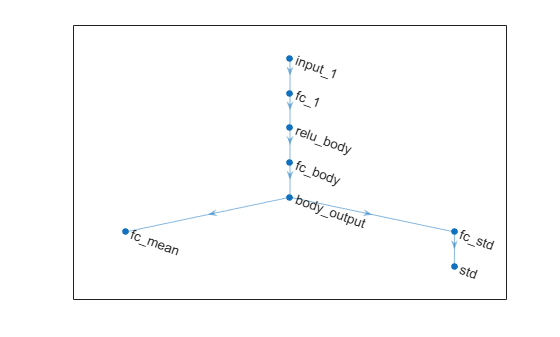# setModel

Set function approximation model for actor or critic

Since R2020b

## Syntax

``newFcnAppx = setModel(oldFcnAppx,model)``

## Description

example

````newFcnAppx = setModel(oldFcnAppx,model)` returns a new actor or critic function object, `newFcnAppx`, with the same configuration as the original function object, `oldFcnAppx`, and the computational model specified in `model`.```

## Examples

collapse all

Create an environment with a continuous action space and obtain its observation and action specifications. For this example, load the environment used in the example Train DDPG Agent to Control Double Integrator System.

`env = rlPredefinedEnv("DoubleIntegrator-Continuous");`

Obtain observation and action specifications.

```obsInfo = getObservationInfo(env); actInfo = getActionInfo(env);```

Create a PPO agent from the environment observation and action specifications. This agent uses default deep neural networks for its actor and critic.

`agent = rlPPOAgent(obsInfo,actInfo);`

To modify the deep neural networks within a reinforcement learning agent, you must first extract the actor and critic function approximators.

```actor = getActor(agent); critic = getCritic(agent);```

Extract the deep neural networks from both the actor and critic function approximators.

```actorNet = getModel(actor); criticNet = getModel(critic);```

The networks are `dlnetwork` objects. To view them using the `plot` function, you must convert them to `layerGraph` objects.

For example, view the actor network.

`plot(layerGraph(actorNet))`To validate a network, use `analyzeNetwork`. For example, validate the critic network.

```analyzeNetwork(criticNet) ```

You can modify the actor and critic networks and save them back to the agent. To modify the networks, you can use the Deep Network Designer app. To open the app for each network, use the following commands.

```deepNetworkDesigner(layerGraph(criticNet)) deepNetworkDesigner(layerGraph(actorNet)) ```

In Deep Network Designer, modify the networks. For example, you can add additional layers to your network. When you modify the networks, do not change the input and output layers of the networks returned by `getModel`. For more information on building networks, see Build Networks with Deep Network Designer.

To validate the modified network in Deep Network Designer, you must click on Analyze for dlnetwork, under the Analysis section. To export the modified network structures to the MATLAB® workspace, generate code for creating the new networks and run this code from the command line. Do not use the exporting option in Deep Network Designer. For an example that shows how to generate and run code, see Create DQN Agent Using Deep Network Designer and Train Using Image Observations.

For this example, the code for creating the modified actor and critic networks is in the `createModifiedNetworks` helper script.

`createModifiedNetworks`

Each of the modified networks includes an additional `fullyConnectedLayer` and `reluLayer` in their main common path. View the modified actor network.

`plot(layerGraph(modifiedActorNet))`After exporting the networks, insert the networks into the actor and critic function approximators.

```actor = setModel(actor,modifiedActorNet); critic = setModel(critic,modifiedCriticNet);```

Finally, insert the modified actor and critic function approximators into the actor and critic objects.

```agent = setActor(agent,actor); agent = setCritic(agent,critic);```

## Input Arguments

collapse all

Original actor or critic function object, specified as one of the following:

To create an actor or critic function object, use one of the following methods.

Function approximation model, specified as one of the following:

• Deep neural network defined as an array of `Layer` objects, a `layerGraph` object, a `DAGNetwork` object, or a `dlnetwork` object. The input and output layers of `model` must have the same names and dimensions as the network returned by `getModel` for the same function object. Here, the output layer is the layer immediately before the output loss layer.

• `rlTable` object with the same dimensions as the table model defined in `newRep`.

• 1-by-2 cell array that contains the function handle for a custom basis function and the basis function parameters.

When specifying a new model, you must use the same type of model as the one already defined in `newRep`.

Note

For agents with more than one critic, such as TD3 and SAC agents, you must call `setModel` for each critic representation individually, rather than calling `setModel` for the array of returned by `getCritic`.

```critics = getCritic(myTD3Agent); % Modify critic networks. critics(1) = setModel(critics(1),criticNet1); critics(2) = setModel(critics(2),criticNet2); myTD3Agent = setCritic(myTD3Agent,critics); ```

## Output Arguments

collapse all

New actor or critic function object, returned as a function object of the same type as `oldFcnAppx`. Apart from the new computational model, `newFcnAppx` is the same as `oldFcnAppx`.

## Version History

Introduced in R2020b

expand all